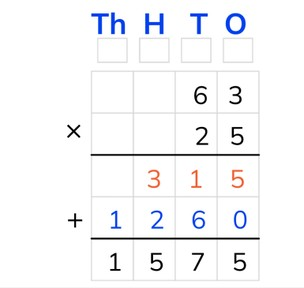Standard algorithm multiplication with two numbers to 100

# Standard algorithm multiplication with two numbers to 100

Students learn standard algorithm multiplication with two numbers to 100.8,000 schools use Gynzy92,000 teachers use Gynzy1,600,000 students use Gynzy

## General

The students learn standard algorithm multiplication with two numbers to 100.

## Standards

CCSS.Math.Content.5.NBT.B.5

## Learning objective

Students will be able to multiply using the standard algorithm with two numbers to 100.

## Introduction

The students solve the problems with a tens number and a number to 100 using the standard algorithm.

## Instruction

Explain that with standard algorithm multiplication you start by putting the numbers one under the other. You put the largest number on top. The T, H, T, O above the table refer to the thousands, hundreds, tens, and ones. Show that above each column there is a regrouping box. In these boxes you can write down numbers that are regrouped to add these to the other outcomes later on. You use the intermediate step in the chart to write down the outcomes of multiplying the ones (O) and tens (T) using the standard algorithm. Next you add these two outcomes together to get the answer to the problem. Explain that you start with the lower number on the right in the ones (O) column. You multiply this number with the higher number from the ones column (5 × 3 = 15). Of this 15, you write the 5 under the first line in the ones column. You can write down the 1 (10) in the regrouping box of the tens. Now multiply the lower number from the ones column with the higher number from the tens column (5 × 6 = 30). Emphasize that you shouldn't forget to add the 1 from the regrouping box of the tens to that (30 + 1 = 31). Now write the 1 with the tens (T) and the 3 with the hundreds (H). This is the first outcome of the intermediate step. Next you multiply the lower number from the tens (T) column with the higher number from the ones (O) column (2 × 3 = 6). Emphasize that with multiplying with a tens number you first write down a 0 in the tens (T) column. Next you multiply the lower number from the tens (T) column with the higher number from the tens (T) column (2 × 6 = 12). Write down the 2 with the hundreds (H) and the 1 with the thousands (T). Emphasize that this is the second outcome of the intermediate step. Finally you add up the two outcomes from the intermediate step together (315 + 1,260 = 1,575). This is the answer to the problem. Explain that with standard algorithm multiplication with a tens number you can directly write down a 0 with the ones, because all multiplication problems with this number make 0. Briefly show that this is referring to the problems 0 × 3 and 0 × 6. Practice two problems that must be solved using standard algorithm multiplication together with the students. Students may use a sheet of scrap paper. The students solve the next three problems on their own. For the first of these the students only have to fill in the intermediate steps and they can check these with the answer given.

Check whether the students can multiply using the standard algorithm with two numbers to 100 by asking the following question:
- Which steps do you use to solve the problem 29 × 26 using the standard algorithm?

## Quiz

The students test their understanding of standard algorithm multiplication with two numbers to 100 through ten exercises. In some exercises the students must fill in the numbers into the chart on their own and then solve the problem, and for other exercises the chart has already been filled in and the students just have to solve the problem.

## Closing

Discuss once again the importance of being able to multiply using the standard algorithm with two numbers to 100. As a closing activity the students can solve a problem in pairs, for which they must provide the answer in smileys. To check the answer the smileys can be dragged onto the chart. As an extra exercise the students can make a smiley problem on their own and have their partner solve it.

## Teaching tips

Have students that have difficulty with standard algorithm multiplication with two numbers to 100 first practice standard algorithm multiplication with one number to 100. Emphasize that the students can use the regrouping boxes.

## Instruction materials

Scrap paper

### The online teaching platform for interactive whiteboards and displays in schools

• Save time building lessons

• Manage the classroom more efficiently

• Increase student engagement